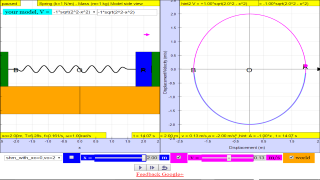SHM09

### 1.2.3 Velocity LO (f)

From x = xo sin ω t

differentiating we get

$v=\genfrac{}{}{0.1ex}{}{dx}{dt}={x}_{0}\omega \left(sin\omega t\right)={v}_{0}cos\left(\omega t\right)$

where   v0 = x0 ω    is the maximum velocity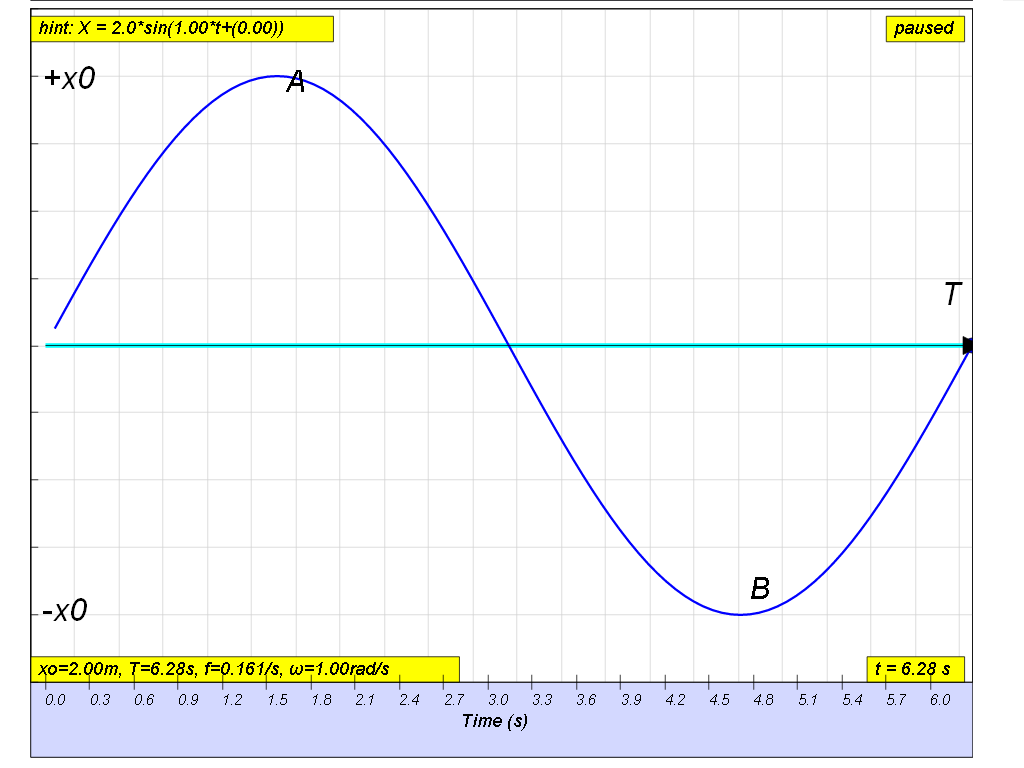Variation with time of velocity

## In terms of x:

From mathematical identity     cos2 ωt + sin2 ωt = 1,

rearranging

cos2 ωt       = 1 - sin2 ωt

$cos\omega t=±\sqrt{\left(1-si{n}^{2}\omega t\right)}$

since

v       =  x0ω cos ωt

where x0 is the maximum displacement

$v=±{x}_{0}\omega \sqrt{\left(1-si{n}^{2}\omega t\right)}$
$v=±{x}_{0}\omega \sqrt{\left(1-\left(\frac{x}{{x}_{0}}{\right)}^{2}\right)}$

$v=±\omega \sqrt{\left({{x}_{0}}^{2}-{x}^{2}\right)}$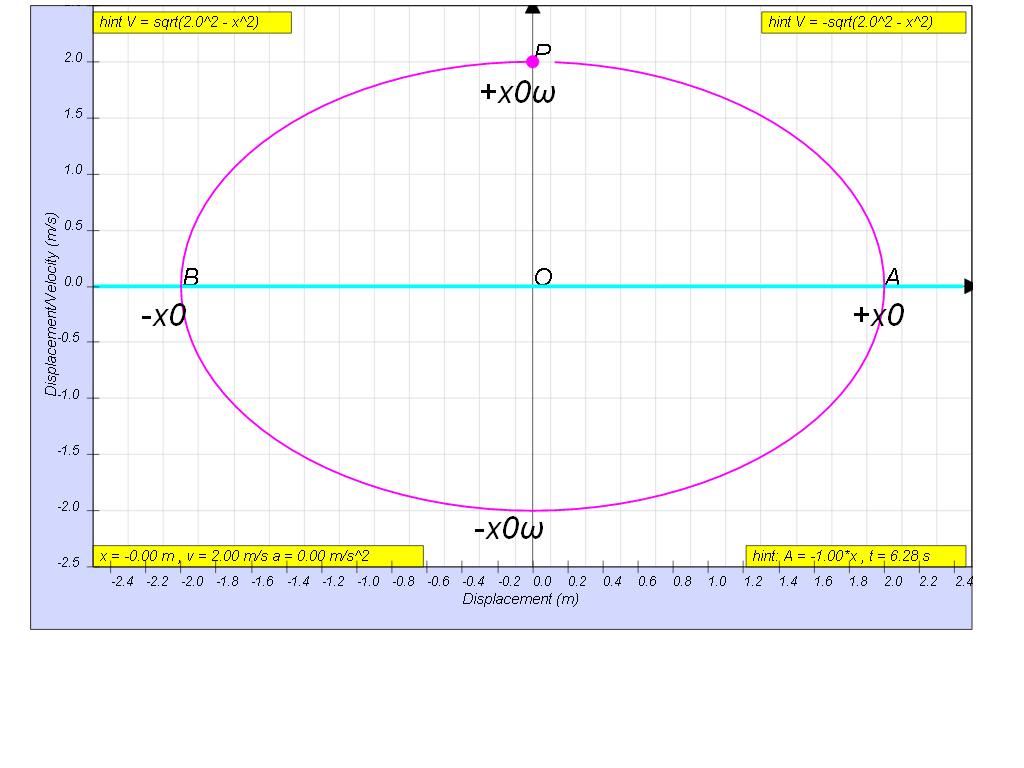Variation with displacement of velocity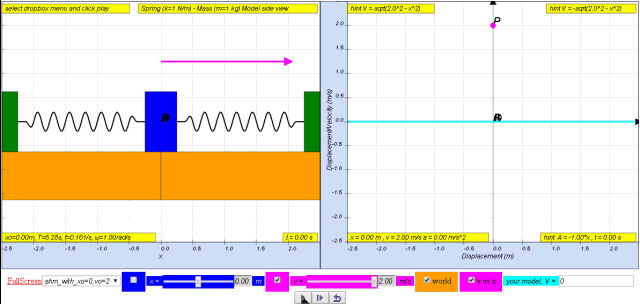### Translations

Code Language Translator Run### Software Requirements

SoftwareRequirements

 Android iOS Windows MacOS with best with Chrome Chrome Chrome Chrome support full-screen? Yes. Chrome/Opera No. Firefox/ Samsung Internet Not yet Yes Yes cannot work on some mobile browser that don't understand JavaScript such as..... cannot work on Internet Explorer 9 and below

### CreditsThis email address is being protected from spambots. You need JavaScript enabled to view it.

### end faq

http://iwant2study.org/lookangejss/02_newtonianmechanics_8oscillations/ejss_model_SHM09/SHM09_Simulation.xhtml

## Apps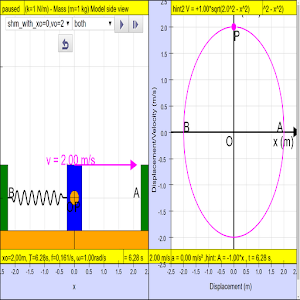https://play.google.com/store/apps/details?id=com.ionicframework.shm09app611123&hl=en

### end faq

3.875 1 1 1 1 1 1 1 1 1 1 Rating 3.88 (4 Votes)